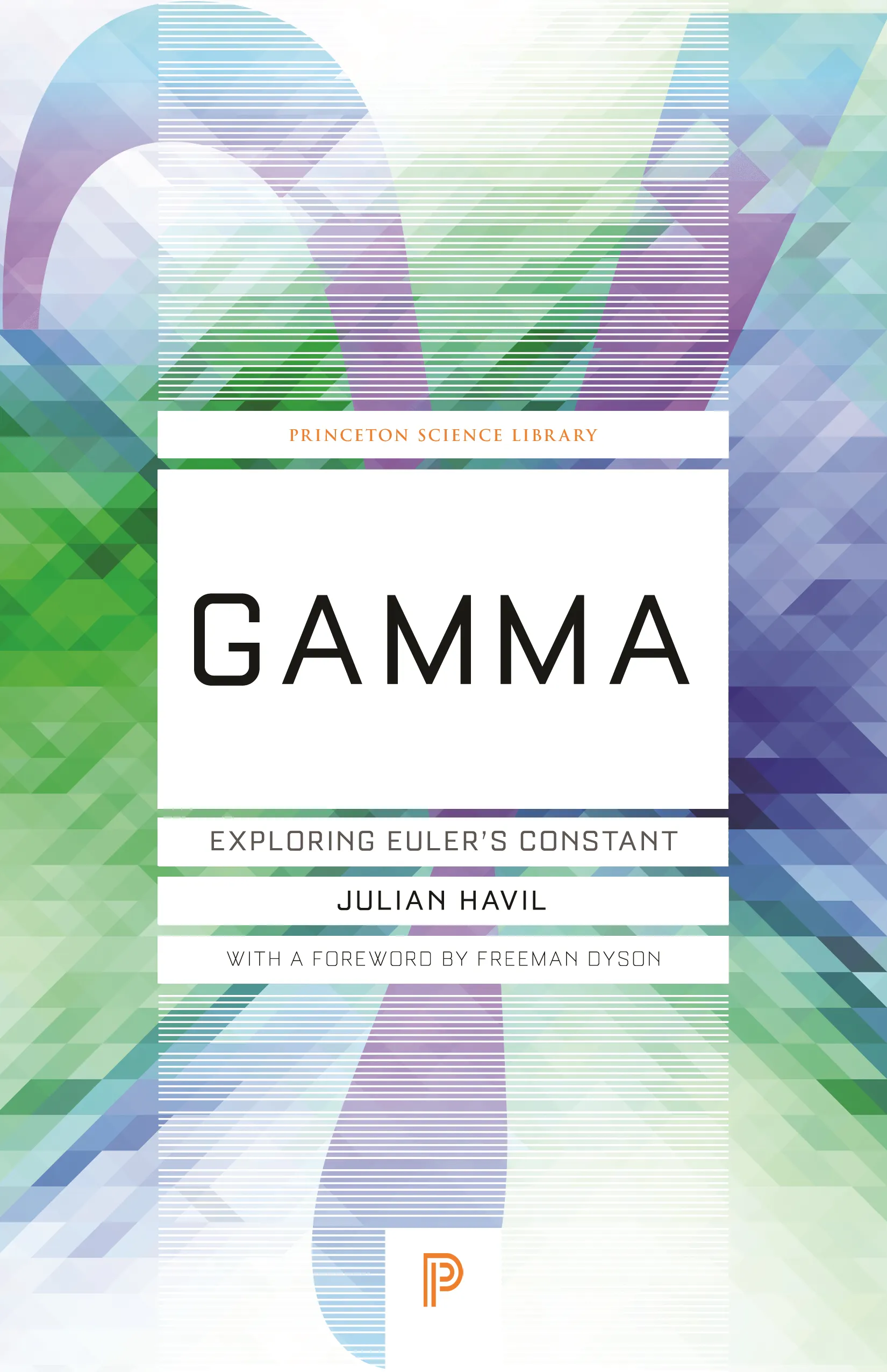#### Gamma

Julian Havil

##### ebooks

Many of our ebooks are available for purchase from these online vendors:

Many of our ebooks are available through library electronic resources including these platforms:

# Gamma: Exploring Euler's Constant

Foreword by
• Freeman Dyson## Paperback

Price:
\$18.95 / £14.99
ISBN:
Published:
Oct 31, 2017
2018
Pages:
296
Size:
5.5 x 8.5 in.
Illus:
89 b/w illus., 20 tables

Among the many constants that appear in mathematics, π, e, and i are the most familiar. Following closely behind is y, or gamma, a constant that arises in many mathematical areas yet maintains a profound sense of mystery.

In a tantalizing blend of history and mathematics, Julian Havil takes the reader on a journey through logarithms and the harmonic series, the two defining elements of gamma, toward the first account of gamma’s place in mathematics.

Introduced by the Swiss mathematician Leonhard Euler (1707-1783), who figures prominently in this book, gamma is defined as the limit of the sum of 1 + 1/2 + 1/3 + … Up to 1/n, minus the natural logarithm of n — the numerical value being 0.5772156… . But unlike its more celebrated colleagues π and e, the exact nature of gamma remains a mystery — we don’t even know if gamma can be expressed as a fraction.

Among the numerous topics that arise during this historical odyssey into fundamental mathematical ideas are the Prime Number Theorem and the most important open problem in mathematics today — the Riemann Hypothesis (though no proof of either is offered!).

Sure to be popular with not only students and instructors but all math aficionados, Gamma takes us through countries, centuries, lives, and works, unfolding along the way the stories of some remarkable mathematics from some remarkable mathematicians.